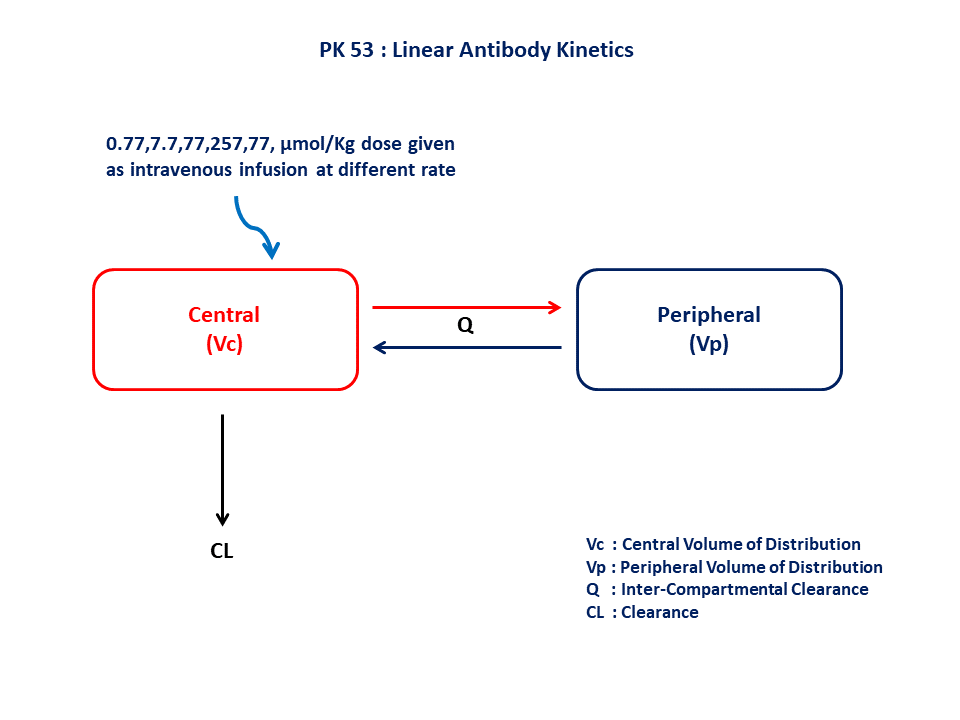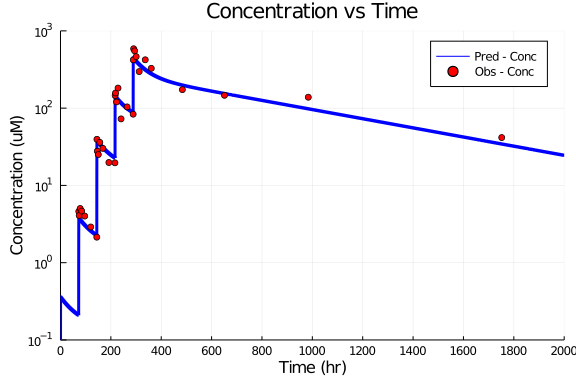# Exercise PK53 - Linear Antibody Kinetics

### Background

Following info:

• Structural model - Two compartment model

• Route of administration - Intravenous infusion

• Dosage Regimen - 0.77,7.7,77,257,771, μmol/Kg dose given as intravenous infusion

• Number of Subjects - 1 (Monkey)### Objectives

In this tutorial, you will learn how to build two compartment turnover model to characterize linear antibody kinetics and simulate the model for one single subject and different dosage regimen.

### Libraries

call the "necessary" libraries to get started

using Pumas
using Plots
using CSV
using StatsPlots
using Random


### Model

In this model, we administer dose in central compartment.

pk_53          = @model begin
@param begin
tvvc       ∈ RealDomain(lower=0)
tvvp       ∈ RealDomain(lower=0)
tvcl       ∈ RealDomain(lower=0)
tvq        ∈ RealDomain(lower=0)
Ω          ∈ PDiagDomain(4)
σ²_prop    ∈ RealDomain(lower=0)
end

@random begin
η          ~ MvNormal(Ω)
end

@pre begin
Vc         = tvvc * exp(η)
Vp         = tvvp * exp(η)
CL         = tvcl * exp(η)
Q          = tvq * exp(η)
end

@dynamics begin
Central'   = -(Q/Vc)*Central +(Q/Vp)*Peripheral -(CL/Vc)*Central
Peripheral'=  (Q/Vc)*Central -(Q/Vp)*Peripheral
end

@derived begin
cp         = @. Central/Vc
dv         ~ @. Normal(cp, sqrt(cp^2*σ²_prop))
end
end

PumasModel
Parameters: tvvc, tvvp, tvcl, tvq, Ω, σ²_prop
Random effects: η
Covariates:
Dynamical variables: Central, Peripheral
Derived: cp, dv
Observed: cp, dv


### Parameters

The parameters are as given below. tv represents the typical value for parameters.

• Cl - Clearance (L/hr/kg)

• Vc - Volume of Central Compartment (L/kg)

• Vp - Volume of Peripheral Compartment (L/kg)

• Q - Intercompartmental CLearance (L/hr/kg)

• Ω - Between Subject Variability

• σ - Residual error

param = (tvvc    = 2.139,
tvvp    = 1.5858,
tvcl    = 0.00541,
tvq     = 0.01640,
Ω       = Diagonal([0.00,0.00,0.00,0.00]),
σ²_prop = 0.04)

(tvvc = 2.139, tvvp = 1.5858, tvcl = 0.00541, tvq = 0.0164, Ω = [0.0 0.0 0.
0 0.0; 0.0 0.0 0.0 0.0; 0.0 0.0 0.0 0.0; 0.0 0.0 0.0 0.0], σ²_prop = 0.04)


### Dosage Regimen

• Dose 1:- 0.77 μmol/kg given as an IV-infusion at time=0

• Dose 2:- 7.7 μmol/kg given as an IV-infusion at time=72.17

• Dose 3:- 77 μmol/kg given as an IV-infusion at time=144.17

• Dose 4:- 257 μmol/kg given as an IV-infusion at time=216.6

• Dose 5:- 771 μmol/kg given as an IV-infusion at time=288.52

ev1  = DosageRegimen(0.77,time=0,cmt=1,duration=0.416667)
ev2  = DosageRegimen(7.7,time=72.17,cmt=1,duration=0.5)
ev3  = DosageRegimen(77,time=144.17,cmt=1,duration=0.5)
ev4  = DosageRegimen(257,time=216.6,cmt=1,duration=0.4)
ev5  = DosageRegimen(771,time=288.52,cmt=1,duration=0.5)
ev   = DosageRegimen(ev1,ev2,ev3,ev4,ev5)
sub1 = Subject(id=1, events=ev)

Subject
ID: 1
Events: 10


### Simulation

Lets simulate for plasma concentration with the specific observation time points after Intravenous administration.

Random.seed!(123)
sim_sub1 = simobs(pk_53,sub1,param,obstimes=0.00:0.01:2000)
df1      = DataFrame(sim_sub1)


### Dataframe & Plot

Use the dataframe for plotting

df1_dv = filter(x -> x.time in [72.67,74.17,78.17,84.17,96.17,120.17,144.17,144.67,146.17,150.17,156.17,168.17,192.17,216.17,217,218.5,222.5,228.5,240.5,264.5,288.5,289.02,290.5,294.5,300.5,312.5,336.5,360.5,483.92,651.25,983.92,1751.92], df1)

@df df1 plot(:time,:cp,yaxis=:log,
title="Concentration vs Time", xlabel="Time (hr)", ylabel="Concentration (uM)",
label="Pred - Conc", linewidth=3, color=:blue,
xticks=[0,200,400,600,800,1000,1200,1400,1600,1800,2000], xlims=(0,2000),
yticks=[0.1,1,10,100,1000],ylims=(0.1,1000))
@df df1_dv scatter!(:time, :dv, label="Obs - Conc", color=[:red])